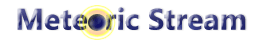# Meteoric Stream - Original Apps & Music, Piano Instrumental, Children music, HymnsJapanese | English
Meteoric Stream -> Documentation -> Python -> [Python] Calculate UPC code check digit

## [Python] Calculate UPC code check digit

```I wrote calculate function for UPC check digit with Python.
UPC code (UPC-A) has below 12 digits:

No.1 : Number System Character
No.2 - 6 : Company Code (5 digits)
No.7 - 11 : Item Code (5 digits)
No.12 : Check Digit

Last of digit is a check digit.

It has mathematical formula that an totals of even number of digits multiplied by 3 plus totals of odd digits, and division remainder of above-mentioned minus 10, and convert to a natural number.

...oops, it's difficult...

Actually, it's very easy when there is code.

def upc_check_digit(number):
return str(10 - ((
int(number) +
int(number) +
int(number) +
int(number) +
int(number) +
int(number)
) * 3 + (
int(number) +
int(number) +
int(number) +
int(number) +
int(number))) % 10)

print(upc_check_digit("12345678901"))

Result: 2 (string)

It's easy.
```

Lastupdated：2017/11/15
Wrote by：2017/11/09